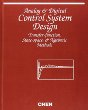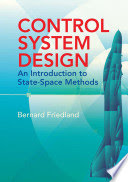Linear Control Systems Design

Course Description

Introduction to linear control system design. Input/output (external) and (internal) state-space mathematical models. Structural characteristics: stability, controllability, observability, canonic forms and minimal realization. State-space controller design by eigenvalue placement. Observer design, total controller, separation principle. Control system analysis via root locus method. Lead- and lag-compensator design with root locus method. Uncertainty modelling; sensitivity and robustness. Controller with two degrees of freedom: polynomial pole placement method, Diophantine equation, disturbance rejection. Optimal control: state-space system stability (Lyapunov stability), continuous-time and discrete-time linear quadratic regulator.

General Competencies

Students will learn how to design linear control systems by various methods.

Learning Outcomes

1. analyze controllability and observability of linear systems
2. design state-space controller and appropriate (deterministic) observer
3. design controller with frequency design methods
4. apply root-locus method for analysis and synthesis
5. apply pole placement controller design approach
6. design linear quadratic regulator for discrete-time systems

Forms of Teaching

Lectures

3 hours of lectures per week.

Exams

Midterm exam and Final exam. During lectures there will be 3 short written tests.

Consultations

After each lecture.

Continuous Assessment Exam
Type Threshold Percent of Grade Threshold Percent of Grade
Quizzes 0 % 15 % 0 % 15 %
Attendance 0 % 5 % 0 % 5 %
Mid Term Exam: Written 0 % 35 % 0 %
Final Exam: Written 0 % 45 %
Exam: Written 0 % 60 %
Exam: Oral 20 %
Comment:

To pass the course student must achieve at least 50 points, of which at least 40 points must be from the midterm exam and final exam (Continuous Assessment) or 30 points from the written exam (Exam).

Week by Week Schedule

1. Introducing control system design problem. Performance indices in time and frequency domain. Mathematical models. Choice of poles and zeros of the closed loop system.
2. Structural characteristics: Stability, controllability, observability, canonic forms and minimal realization.
3. State-space regulator.
4. Time-optimal control (dead-beat controller). Disturbance compensation - introduction of integral action in the control loop.
5. Full order observer. State-space control with observer in the control loop.
6. Frequency methods of controller design.
7. Frequency methods of controller design.
8. Midterm exam.
9. Root locus method. System analysis via root-locus method.
10. Control system synthesis via root-locus method.
11. Pole placement.
12. Pole placement.
13. Linear quadratic regulator (LQR) for discrete-time systems. Mathematical background
14. LQR with infinite horizon. LQR for continuous-time systems. LQR for reference tracking.
15. Final exam.

Study Programmes

Control Engineering and Automation (profile)
Theoretical Course (1. semester)

LiteratureC. T. Chen (1993.), Analog & Digital Control System Design: Transfer Function, State-space & Algebraic Methods, Saunders College PublishingBernard Friedland (1986.), Control System Design: An introduction to State Space Methods, McGraw Hill
Zoran Vukić, Ljubomir Kuljača (2005.), Automatsko upravljanje - analiza linearnih sustava, Kigen d.o.o. Zagreb
Mato Baotić (2016.), Sinteze linearnih sustava upravljanja, Sveučilište u Zagrebu Fakultet elektrotehnike i računarstva

General

ID 34365
Winter semester
5 ECTS
L1 English Level
L1 e-Learning
45 Lectures
0 Exercises
0 Laboratory exercises
0 Project laboratory